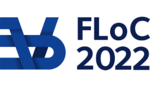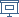FLOC 2022: FEDERATED LOGIC CONFERENCE 2022
SAT ON WEDNESDAY, AUGUST 3RD
Days:
previous day
next day
all days

View: session overviewtalk overviewside by side with other conferences

08:30-09:00Coffee & Refreshments
09:00-10:30 Session 56F: QBF-2 + Stochastic Boolean Satisfiability
09:00
Quantified CDCL with Universal Resolution

ABSTRACT. Quantified Conflict-Driven Clause Learning (QCDCL) solvers for QBF generate Q-resolution proofs. Pivot variables in Q-resolution must be existentially quantified. Allowing resolution on universally quantified variables leads to a more powerful proof system called QU-resolution, but so far, QBF solvers have used QU-resolution only in very limited ways. We present a modified version of QCDCL that generates proofs in QU-resolution by leveraging propositional unit propagation. Experiments with crafted instances and benchmarks from recent QBF evaluations demonstrate the viability of this approach.

09:30
Relating existing powerful proof systems for QBF
PRESENTER: Leroy Chew

ABSTRACT. We advance the theory of QBF proof systems by showing the first simulation of the universal checking format QRAT by a theory-friendly system. We show that the sequent system G fully p-simulates QRAT, including the Extended Universal Reduction (EUR) rule which was recently used to show QRAT does not have strategy extraction. Because EUR heavily uses resolution paths our technique also brings resolution path dependency and sequent systems closer together. While we do not recommend G for practical applications this work can potentially show what features are needed for a new QBF checking format stronger than QRAT.

10:00
Quantifier Elimination in Stochastic Boolean Satisfiability
PRESENTER: Hao-Ren Wang

ABSTRACT. Stochastic Boolean Satisfiability (SSAT) generalizes quantified Boolean formulas (QBFs) by allowing quantification over random variables. Its generality makes SSAT powerful to model decision or optimization problems under uncertainty. On the other hand, this generalization complicates the computation in its counting nature. In this work, we address the following two questions: 1) Is there an analogy of quantifier elimination in SSAT, similar to QBF? 2) If quantifier elimination is possible for SSAT, can it be effective for SSAT solving? The answers are affirmative. We develop an SSAT decision procedure based on quantifier elimination. Experimental results demonstrate the unique benefits of the new method compared to the state-of-the-art solvers.

10:30-11:00Coffee Break
11:00-12:30 Session 58H: Applications + pragmatics
11:00
A SAT Attack on Rota's Basis Conjecture

ABSTRACT. The SAT modulo Symmetries (SMS) is a recently introduced framework for dynamic symmetry breaking in SAT instances. It combines a CDCL SAT solver with an external lexicographic minimality checking algorithm.

We extend SMS from graphs to matroids and use it to progress on Rota's Basis Conjecture (1989), which states that one can always decompose a collection of r disjoint bases of a rank r matroid into r disjoint rainbow bases. Through SMS, we establish that the conjecture holds for all matroids of rank 4 and certain special cases of matroids of rank 5. Furthermore, we extend SMS with the facility to produce DRAT proofs. External tools can then be used to verify the validity of additional axioms produced by the lexicographic minimality check.

As a byproduct, we have utilized our framework to enumerate matroids modulo isomorphism and to support the investigation of various other problems on matroids.

11:30
The packing chromatic number of the infinite square grid is at least 14

ABSTRACT. A k-packing coloring of a graph G = (V, E) is a mapping from V to {1, ..., k} such that any pair of vertices u, v that receive the same color i must be at distance greater than i in G. Arguably the most fundamental problem regarding packing colorings is to determine the packing chromatic number of the infinite square grid. A sequence of previous works has proved this number to be between 13 and 15. Our work improves the lower bound to 14. Moreover, we present a new encoding that is asymptotically more compact than the previously used ones.

12:00
PRESENTER: Benjamin Kiesl

ABSTRACT. We present approaches to store and restore the state of a SAT solver, allowing us to migrate the state between different compute resources, or even between different solvers. This can be used in many ways, e.g., to improve the fault tolerance of solvers, to schedule SAT problems on a restricted number of cores, or to use dedicated preprocessing tools for inprocessing. We identify a minimum viable subset of the solver state to migrate such that the loss of performance is small. We then present and implement two different approaches to state migration: one approach stores the state at the end of a solver run whereas the other approach stores the state continuously as part of the proof trace. We show that our approaches enable the generation of correct models and valid unsatisfiability proofs. Experimental results confirm that the overhead is reasonable and that in several cases solver performance actually improves.

12:30-14:00Lunch Break

Lunch will be held in Taub lobby (CP, LICS, ICLP) and in The Grand Water Research Institute (KR, FSCD, SAT).

14:00-15:30 Session 59G: Theory
14:00
Tight Bounds for Tseitin Formulas
PRESENTER: Petr Smirnov

ABSTRACT. We show that for any connected graph G the size of any regular resolution or OBDD(and, reordering) refutation of a Tseitin formula based on G is at least 2^Omega(tw(G)), where tw(G) is the treewidth of G. These lower bounds improve upon the previously known bounds and, moreover, they are tight.

For both of the proof systems, there are constructive upper bounds that almost match the obtained lower bounds, hence the class of Tseitin formulas is almost automatable for regular resolution and for OBDD(and, reordering).

14:30
A generalization of the Satisfiability Coding Lemma and its applications
PRESENTER: Harry Sha

ABSTRACT. The seminal Satisfiability Coding Lemma of Paturi, Pudlák, and Zane is a coding scheme for satisfying assignments of $k$-CNF formulas. We generalize it to give a coding scheme for implicants and use this generalized scheme to establish new structural and algorithmic properties of prime implicants of $k$-CNF formulas.

Our first application is a near-optimal bound of $n \cdot 3^{n(1-\Omega(1/k))}$ on the number of prime implicants of any $n$-variable $k$-CNF formula. This resolves an open problem from the Ph.D. thesis of Talebanfard, who proved such a bound for the special case of constant-read $k$-CNF formulas. Our proof is algorithmic in nature, yielding an algorithm for computing the set of all prime implicants---the Blake Canonical Form---of a given $k$-CNF formula. The problem of computing the Blake Canonical Form of a given function is a classic one, dating back to Quine, and our work gives the first non-trivial algorithm for $k$-CNF formulas.

15:00
On the Parallel Parameterized Complexity of MaxSAT Variants
PRESENTER: Max Bannach

ABSTRACT. In the maximum satisfiability problem (\textsc{max-sat}) we are given a propositional formula in conjunctive normal form and have to find an assignment that satisfies as many clauses as possible. We study the \emph{parallel} parameterized complexity of various versions of \textsc{max-sat} and provide the first \emph{constant-time} algorithms parameterized either by the solution size or by the allowed excess relative to some guarantee (above guarantee'' versions). For the \emph{dual parameterized} version where the parameter is the number of clauses we are allowed to leave unsatisfied, we present the first parallel algorithm for \textsc{max-2sat} (known as \textsc{almost-2sat}). The difficulty in solving \textsc{almost-2sat} in \emph{parallel} comes from the fact that the iterative compression method, originally developed to prove that the problem is fixed-parameter tractable at all, is inherently \emph{sequential.} We observe that a graph flow whose value is a parameter can be computed in parallel and use this fact to develop a parallel algorithm for the vertex cover problem parameterized above the size of a given matching. Finally, we study the parallel complexity of \textsc{max-sat} parameterized by the vertex cover number, the treedepth, the feedback vertex set number, and the treewidth of the input's incidence graph. While \textsc{max-sat} is fixed-parameter tractable for all of these parameters, we show that they allow different degrees of possible parallelization. For all four we develop dedicated parallel algorithms that are \emph{constructive}, meaning that they output an optimal assignment~--~in contrast to results that can be obtained by parallel meta-theorems, which often only solve the decision version.

15:30-16:00Coffee Break
16:00-17:30 Session 61H: Model Counting
16:00
Proofs for Propositional Model Counting
PRESENTER: Markus Hecher

ABSTRACT. Although propositional model counting (#SAT) was long considered too hard to be practical, today’s highly efficient solvers facilitate applications in probabilistic reasoning, reliability estimation, quantitative design space exploration, and more. The current trend of solvers growing more capable every year is likely to continue as a diverse range of algorithms are explored in the field. However, to establish model counters as reliable tools like SAT-solvers, correctness is as critical as speed. As in the nature of complex systems, bugs emerge as soon as the tools are widely used. To identify and avoid bugs, explain decisions, and provide trustworthy results, we need verifiable results. In this paper, we propose a novel proof system for certifying model counts. We show how proof traces can be generated efficiently for exact model counters based on dynamic programming, counting CDCL with component caching, as well as knowledge compilation to d-DNNF. These approaches are the most widely used techniques in exact implementations. Furthermore, we provide proof-of-concept software for emitting proofs from model counters and a parallel trace checker. Based on this, we show the feasibility of using certified model counting in an empirical experiment.

16:30
A New Exact Solver for (Weighted) Max#SATPRESENTER: Marie Miceli

ABSTRACT. We present and evaluate d4Max, an exact approach for solving the Weighted Max#SAT problem. The Max#SAT problem extends the model counting problem (#SAT) by considering a tripartition of the variables {X, Y, Z}, and consists in maximizing over the variables X the number of assignments to Y that can be extended to a solution with some assignment to Z. The weighted Max#SAT problem is an extension of the Max\SAT problem with weights associated on each interpretation. We test and compare our approach with other state-of-the-art solvers on the challenging task in probabilistic inference of finding the marginal maximum a posteriori probability (MMAP) of a given subset of the variables in a Bayesian network and on exist-random quantified SSAT benchmarks. The results clearly show the overall superiority of d4Max in term of speed and number of instances solved. Moreover, we experimentally show that, in general, d4Max is able to quickly spot a solution that is close to optimal, thereby opening the door to an efficient anytime approach.

17:00
Weighted Model Counting with Twin-Width
PRESENTER: Stefan Szeider

ABSTRACT. Bonnet et al. (FOCS 2020) introduced the graph invariant twin-width and showed that many NP-hard problems are tractable for graphs of bounded twin-width, generalizing similar results for other width measures including treewidth and clique-width. In this paper, we investigate the use of twin-width for solving the propositional satisfiability problem (SAT) and model counting. We particularly focus on Bounded-ones Weighted Model Counting (BWMC), which takes as input a CNF formula F along with a bound k and asks for the weighted sum of all models with at most k positive literals. BWMC generalizes not only SAT, but also (weighted) model counting.

We develop the notion of "signed" twin-width of CNF formulas, and establish that BWMC is fixed-parameter tractable when parameterized by the certified signed twin-width of F plus k. We show that this result is tight: it is neither possible to drop the bound k or use the vanilla twin-width instead if one wishes to retain fixed-parameter tractability, even for the easier problem SAT. Our theoretical results are complemented with an empirical evaluation and comparison of signed twin-width on various classes of CNF formulas.

18:30-20:30 Walking tour (at Haifa)

pickup at 18:00 from the Technion (Tour at Haifa, no food will be provided)